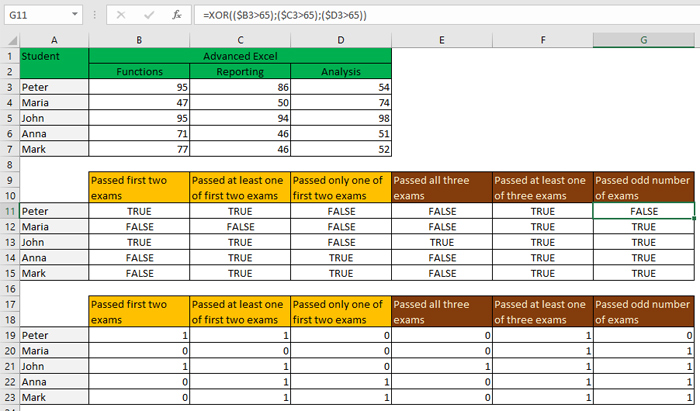# Student performance analysis

With the exception of the IF function, Excel has multitude of logical functions that are often unfairly neglected. The AND, OR, and XOR functions are also used to test the one or more conditions. The first returns TRUE if all conditions are met, the second if at least one of the conditions is met, and the third is used to examine cases where at least one but not all conditions are met. These functions can be used to analyze student performance on exams.

Since I plan to tryout at online courses soon, I chose a suitable topic: example is a table that shows the number of points that 5 students won in 3 exams. The exams of course relate to the Masterclass courses I intend to teach (although, in reality, course participants solve exercises and do homework; formally, there is no final exam). In order for a student to pass the exam, he must to earn more than 65 points.

If we want to know how many students have passed the first two exams we should write the formula:

=AND((\$B3>65),(\$C3>65))

Only if both conditions are met (the student has passed both exams) Excel returns the value TRUE. Otherwise, it returns FALSE. If we wanted to know if student passed at least one of the first two exams, the formula would be:

=OR((\$B3>65),(\$C3>65))

And if we want to know if student has passed at least one of the first two exams, but not both of them, the formula is:

=XOR((\$B3>65),(\$C3>65))

In the formulas, we could set more than two conditions. With the AND and OR functions nothing changes while the XOR function returns TRUE exclusively for an odd number of correct statements.

How to get numbers instead of TRUE and FALSE? Very simply, a “double negation” should be used:

=–AND((\$B3>65),(\$C3>65))

As a result, 0 will appear in the cell where the value was FALSE and 1 where the value was TRUE. The values ​​expressed in this way are easier to summarize or count, which may be useful for further analysis.